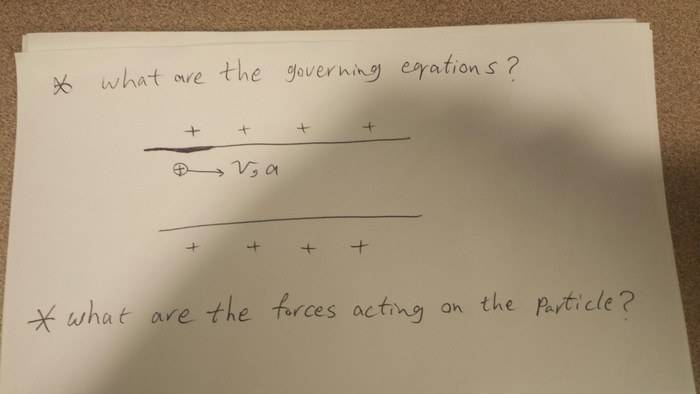# Electric Field between two plates with the same charge

Hi

I'm looking for a equation (or equations) needed to calculate the electric field between to plates with the same charge. For example, two plates with positive charge.

Any help is appreciated.

Calculating electric field strength between the plates? No field lines from either plate will terminate on the other. Field lines are between surfaces of different charge only.

Being able to draw the field of both plates? I don't think there are equations, just guidelines for understanding. All lines begin perpendicular and can not intersect.

Thanks for your answer. Actually I'm not really familiar with these concept. So sorry if my questions look really basic.

So, like you said, we don't have any field. now, imagine a positively charged particle passes through two positively charged plates. How can I write the energy balance for the motion of particle?

Thanks.

Oh man, you're going down a path I avoid like the plague. I hate those equations. First, you will have to determine the speed the particle is moving for it to have the energy to move towards two like charged plates.

Could you briefly give me some hints (and also names) of those equations? I think I need to solve some ODEs, I'm familiar with kinematic part (yes! mechanical engineer!) but honestly, I have no clue about the electrical part. :)

Thanks

Thanks Samon4.

This is the problem ( V is velocity, "a" is acceleration)Khashishi
If there's nothing else in the universe, there's no field between two plates with the same charge.

Redbelly98
Staff Emeritus